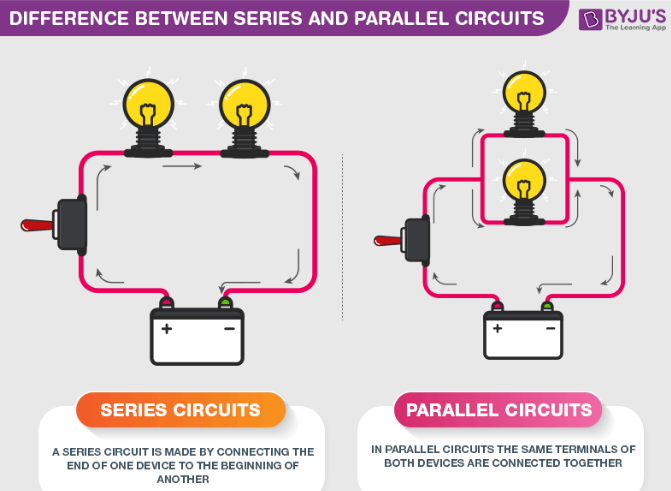# MCQs on Series and Parallel Circuits

Series Circuit: The components are connected in end-to-end in a series circuit. The current will remain the same in all parts of the circuit. In a closed series circuit, there will be only one path of the current. The Voltage flow is different across each component.

Parallel Circuit: The components in a parallel circuit are connected across each other. The current flow differs through each component of the circuit whereas the voltage remains the same.Q1: A certain circuit is composed of two parallel resistors. The total resistance is 1,403 Ω. One of the resistors is 2 Ω. The other resistor value is

1. 1,403 Ω
2. 4.7 kΩ
3. 2 kΩ
4. 3,403 Ω

Q2: A voltage divider consists of ot two 100 kΩ resistors and a 12 V source. What will the voltage be if a load resistor of 1 MΩ is connected to the output?

1. 0.57 V
2. 6 V
3. 12 V
4. 5.7 V

Q3: The parallel combination of a 1.5 kΩ resistor and 470 Ω resistor is in series with the parallel combination of five 1 kΩ resistors. The source voltage is 50 V. The percentage of the load current through any single 1 kΩresistor is

1. 25 %
2. 20 %
3. 100 %
4. 50 %

Q4: On which of the following voltage range settings will a voltameter present minimum load on a circuit?

1. 1 V
2. 50 V
3. 500 V
4. 1,000 V

Q5: A Voltage divider consists of two 68 kΩ resistors and a 24 V source. The unknown output voltage is

1. 12 V
2. 24 V
3. 0 V
4. 6 V

Q6: Two 3.3 kΩ resistors are in series combination are in parallel with a 4.7 kΩ resistor. What will be the voltage across the 4.7 kΩ resistors if the voltage across one of the 3.3 kΩ resistors is 12 V

1. 24 V
2. 12 V
3. 0 V
4. 6 V

Q7: The 1.2 kΩ resistors are in series and this series combination is in parallel with a 3.3 3.3 kΩ resistor. The total resistance is

1. 138 Ω
2. 1,389 Ω
3. 5,700 Ω
4. 880 Ω

Q8: A certain voltage divider consists of three 1 kΩ resistors in series. Which of the following load resistors will have the least effect on the output voltage?

1. 1 MΩ
2. 100 kΩ
3. 1 kΩ
4. 330 Ω

Q9: A certain Wheatstone bridge has the following resistor values: R1= 10 kΩ, R2 = 720 Ω and R4 = 2.4 kΩ. The unknown resistance is

1. 24 Ω
2. 2.4 Ω
3. 300 Ω
4. 3,000 Ω